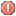View Single Post
#1 (permalink)dsgdsg Guest Posts: n/aVery slow Import XLSX file to access MDB

Hello there !
I wrote the following code to import an excel table containing some 25 columns and thousands of records.

The import time is extremely long with a few thousands records ... and since the xlsx files might grow and reach 100.000 records ... it will not be acceptable !

Would you have any better method to access the xlsx records and copy them to the MS-access table ?! Many thanks !!

cfile = xPath + "sales.xlsx"
objExcel.Workbooks.Open cfile
nindex = 1

StatusBar.Panels(1) = "Importing from excel ..."

Do While Not (IsNull(objExcel.Cells(nindex, 1)) Or IsEmpty(objExcel.Cells(nindex, 1)))

For X = 1 To UBound(aFields)
If IsNull(objExcel.Cells(nindex, aFields(X).ColumnNumber + 1)) Or _
IsEmpty(objExcel.Cells(nindex, aFields(X).ColumnNumber + 1)) Or _
Len(Trim(objExcel.Cells(nindex, aFields(X).ColumnNumber + 1))) = 0
Then
T_ECSALES.Recordset(aFields(X).AccessFieldName) = 0
Else
T_ECSALES.Recordset(aFields(X).AccessFieldName) = objExcel.Cells (nindex, aFields(X).ColumnNumber + 1)
End If
Next

T_ECSALES.Recordset.Update

nindex = nindex + 1
ProgressBarSALESImport.Value = nindex

Loop

objExcel.Quit
Set objExcel = Nothing